#C# 类型基础

0
0
01. 云栖社区>
2. 博客>
3. 正文

# C# 类型基础

## 值类型 和 引用类型

NOTE：堆栈(stack)是一种后进先出的数据结构，在内存中，变量会被分配在堆栈上来进行操作。堆(heap)是用于为类型实例(对象)分配空间的内存区域，在堆上创建一个对象，会将对象的地址传给堆栈上的变量(反过来叫变量指向此对象，或者变量引用此对象)。

### 1.值类型

public struct ValPoint {
public int x;

public ValPoint(int x) {
this.x = x;
}
}

ValPoint vPoint1;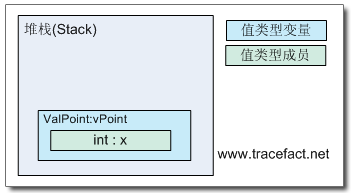NOTE：如果观察MSIL代码，会发现此时变量还没有被压到栈上，因为.maxstack(最高栈数) 为0。并且没有看到入栈的指令，这说明只有对变量进行操作，才会进行入栈。

vPoint1.x = 10;
Console.WriteLine(vPoint.x); // 输出 10

NOTE：如果vPoint1是一个引用类型(比如class)，在运行时会抛出NullReferenceException异常。因为vPoint是一个值类型，不存在引用，所以永远也不会抛出NullReferenceException。

int i;
Console.WriteLine(i);

ValPoint vPoint1 = new ValPoint();
Console.WriteLine(vPoint.x); // 输出为0

• 左边 ValPoint vPoint1，在堆栈上创建一个ValPoint类型的变量vPoint，结构的所有成员均未赋值。在进行new ValPoint()之前，将vPoint压到栈上。
• 右边new ValPoint()，new 操作符不会分配内存，它仅仅调用ValPoint结构的默认构造函数，根据构造函数去初始化vPoint结构的所有字段。

Console.WriteLine((new ValPoint()).x);     // 正常，输出为0

.locals init ( valuetype Prototype.ValPoint CS\$0\$0000) // 声明临时变量
IL_0000:  nop
IL_0001:  ldloca.s   CS\$0\$0000       // 将临时变量压栈
IL_0003:  initobj    Prototype.ValPoint     // 初始化此变量

.locals init ( valuetype Prototype.ValPoint vPoint)       // 声明vPoint
IL_0000:  nop
IL_0001:  ldloca.s   vPoint          // 将vPoint压栈
IL_0003:  initobj    Prototype.ValPoint     // 使用initobj初始化此变量

.locals init ( valuetype Prototype.ValPoint vPoint)
IL_0000:  nop
IL_0001:  ldloca.s   vPoint      // 将 vPoint 压栈
IL_0003:  ldc.i4.s   10          // 将 10 压栈
// 调用构造函数，传递参数
IL_0005:  call       instance void Prototype.ValPoint::.ctor(int32)

### 2.引用类型

public class RefPoint {
public int x;

public RefPoint(int x) {
this.x = x;
}
public RefPoint() {}
}

RefPoint rPoint1;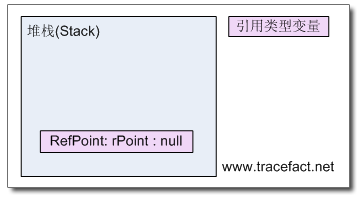rPoint1= new RefPoint(1);

1. 在应用程序堆(Heap)上创建一个引用类型(Type)的实例(Instance)或者叫对象(Object)，并为它分配内存地址。
2. 自动传递该实例的引用给构造函数。(正因为如此，你才可以在构造函数中使用this来访问这个实例。)
3. 调用该类型的构造函数。
4. 返回该实例的引用(内存地址)，赋值给rPoint变量。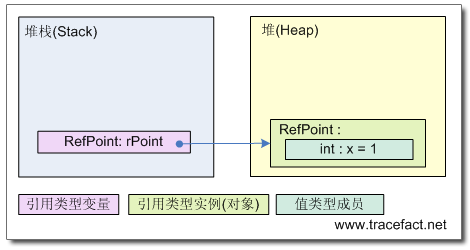### 3.关于简单类型

int i = 3;
int j = 3;
if(i==j) Console.WriteLine("i equals to j");

string a = "123456"; string b = "123456";
if(a == b) Console.WriteLine("a Equals to b");

## 装箱 和 拆箱

1. 在堆上为新生成的对象(该对象包含数据，对象本身没有名称)分配内存。
2. 将 堆栈上 值类型变量的值拷贝到 堆上的对象 中。
3. 将堆上创建的对象的地址返回给引用类型变量(从程序员角度看，这个变量的名称就好像堆上对象的名称一样)。

int i = 1;
Object boxed = i;
Console.WriteLine("Boxed Point: " + boxed);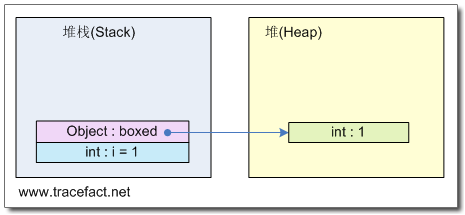MSIL代码是这样的：

.method private hidebysig static void  Main(string[] args) cil managed
{
.entrypoint
// 代码大小       19 (0x13)
.maxstack  1                   // 最高栈数是1，装箱操作后i会出栈
.locals init ( int32 i,     // 声明变量 i(第1个变量，索引为0)
 object boxed)          // 声明变量 boxed (第2个变量，索引为1)
IL_0000:  nop
IL_0001:  ldc.i4.s   10         //#1 将10压栈
IL_0003:  stloc.0                  //#2 10出栈，将值赋给 i
IL_0004:  ldloc.0                  //#3 将i压栈
IL_0005:  box   [mscorlib]System.Int32   //#4 i出栈，对i装箱(复制值到堆，返回地址)
IL_000a:  stloc.1           //#5 将返回值赋给变量 boxed
IL_000b:  ldloc.1           // 将 boxed 压栈
// 调用WriteLine()方法
IL_000c:  call       void [mscorlib]System.Console::WriteLine(object)
IL_0011:  nop
IL_0012:  ret
} // end of method Program::Main

int i = 1;
Object boxed = i;
int j;
j = (int)boxed;          // 显示声明 拆箱后的类型
Console.WriteLine("UnBoxed Point: " + j);

1. 获取已装箱的对象的地址。
2. 将值从堆上的对象中拷贝到堆栈上的值变量中。

## 对象判等

NOTE：有机会较深入地研究这部分内容，需要感谢 微软的开源 以及 VS2008 的FCL调试功能。关于如何调试 FCL 代码，请参考 Configuring Visual Studio to Debug .NET Framework Source Code

public class RefPoint {      // 定义一个引用类型
public int x;
public RefPoint(int x) {
this.x = x;
}
}

public struct ValPoint { // 定义一个值类型
public int x;
public ValPoint(int x) {
this.x = x;
}
}

### 1.引用类型判等

public static bool ReferenceEquals (Object objA, Object objB)
{
return objA == objB;     // #1
}

public virtual bool Equals(Object obj)
{
return InternalEquals(this, obj);    // #2
}

public static bool Equals(Object objA, Object objB) {
if (objA==objB) {        // #3
return true;
}

if (objA==null || objB==null) {
return false;
}

return objA.Equals(objB); // #4
}

// 复制对象引用
bool result;
RefPoint rPoint1 = new RefPoint(1);
RefPoint rPoint2 = rPoint1;

result = (rPoint1 == rPoint2);      // 返回 true;
Console.WriteLine(result);

result = rPoint1.Equals(rPoint2);   // #2 返回true;
Console.WriteLine(result);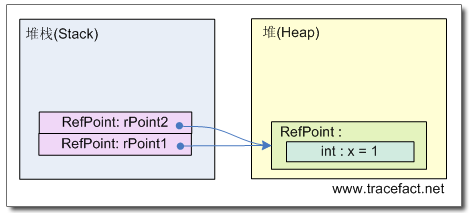//创建新引用类型的对象，其成员的值相等
RefPoint rPoint1 = new RefPoint(1);
RefPoint rPoint2 = new RefPoint(1);

result = (rPoint1 == rPoint2);
Console.WriteLine(result);      // 返回 false;

result = rPoint1.Equals(rPoint2);
Console.WriteLine(result);      // #2 返回false

### 2.简单值类型判等

public override bool Equals (Object obj) {
if (null==obj) {
return false;
}
RuntimeType thisType = (RuntimeType)this.GetType();
RuntimeType thatType = (RuntimeType)obj.GetType();

if (thatType!=thisType) { // 如果两个对象不是一个类型，直接返回false
return false;
}

Object thisObj = (Object)this;
Object thisResult, thatResult;

if (CanCompareBits(this))                // #5
return FastEqualsCheck(thisObj, obj);    // #6

// 利用反射获取值类型所有字段
FieldInfo[] thisFields = thisType.GetFields(BindingFlags.Instance | BindingFlags.Public | BindingFlags.NonPublic);
// 遍历字段，进行字段对字段比较
for (int i=0; i<thisFields.Length; i++) {
thisResult = ((RtFieldInfo)thisFields[i]).InternalGetValue(thisObj,false);
thatResult = ((RtFieldInfo)thisFields[i]).InternalGetValue(obj, false);

if (thisResult == null) {
if (thatResult != null)
return false;
}
else
if (!thisResult.Equals(thatResult)) {  // #7
return false;
}
}

return true;
}

// 复制结构变量
ValPoint vPoint1 = new ValPoint(1);
ValPoint vPoint2 = vPoint1;

result = (vPoint1 == vPoint2);  //编译错误：不能在ValPoint上应用 "==" 操作符
Console.WriteLine(result);

result = Object.ReferenceEquals(vPoint1, vPoint2); // 隐式装箱，指向了堆上的不同对象
Console.WriteLine(result);          // 返回false

Object boxPoint1 = vPoint1;
Object boxPoint2 = vPoint2;
result = (boxPoint1 == boxPoint2);      // 返回false
Console.WriteLine(result);

result = vPoint1.Equals(vPoint2);       // #5 返回true; #6 返回true;
Console.WriteLine(result);      // 输出true

vPoint2.x = 2;
result = vPoint1.Equals(vPoint2);       // #5 返回true; #6 返回false;
Console.WriteLine(result);

## 3. 复杂值类型判等

/* 结构类型 ValLine 的定义，
public struct ValLine {
public RefPoint rPoint;       // 引用类型成员
public ValPoint vPoint;       // 值类型成员
public Line(RefPoint rPoint, ValPoint vPoint) {
this.rPoint = rPoint;
this.vPoint = vPoint;
}
}
*/

RefPoint rPoint = new RefPoint(1);
ValPoint vPoint = new ValPoint(1);

ValLine line1 = new ValLine (rPoint, vPoint);
ValLine line2 = line1;

result = line1.Equals(line2);   // 此时已经存在一个装箱操作，调用ValueType.Equals()
Console.WriteLine(result);      // 返回True

NOTE：进行字段对字段的一对一比较，需要用到反射，如果不了解反射，可以参看 .Net 中的反射 系列文章。

public override bool Equals (Object obj) {

if (CanCompareBits(this))                // #5
return FastEqualsCheck(thisObj, obj);    // #6
// 利用反射获取类型的所有字段(或者叫类型成员)
FieldInfo[] thisFields = thisType.GetFields(BindingFlags.Instance | BindingFlags.Public | BindingFlags.NonPublic);
// 遍历字段进行比较
for (int i=0; i<thisFields.Length; i++) {
thisResult = ((RtFieldInfo)thisFields[i]).InternalGetValue(thisObj,false);
thatResult = ((RtFieldInfo)thisFields[i]).InternalGetValue(obj, false);

if (thisResult == null) {
if (thatResult != null)
return false;
}
else
if (!thisResult.Equals(thatResult)) {  #7
return false;
}
}

return true;
}

1. 进入 ValueType 上的 Equals() 方法，#5 处返回了 false;
2. 进入 for 循环，遍历字段。
3. 第一个字段是RefPoint引用类型，#7 处，调用 System.Object 的Equals()方法，到达#2，返回true。
4. 第二个字段是ValPoint值类型，#7 处，调用 System.ValType的Equals()方法，也就是当前方法本身。此处递归调用。
5. 再次进入 ValueType 的 Equals() 方法，因为 ValPoint 为简单值类型，所以 #5 CanCompareBits 返回了true，接着 #6 FastEqualsCheck 返回了 true。
6. 里层 Equals()方法返回 true。
7. 退出 for 循环。
8. 外层 Equals() 方法返回 true。

## 对象复制

### 1.浅度复制

// 将要进行 浅度复制 的对象，注意为 引用类型
public class RefLine {
public RefPoint rPoint;
public ValPoint vPoint;
public Line(RefPoint rPoint,ValPoint vPoint){
this.rPoint = rPoint;
this.vPoint = vPoint;
}
}
// 定义一个引用类型成员
public class RefPoint {
public int x;
public RefPoint(int x) {
this.x = x;
}
}
// 定义一个值类型成员
public struct ValPoint {
public int x;
public ValPoint(int x) {
this.x = x;
}
}

RefPoint rPoint = new RefPoint(1);
ValPoint vPoint = new ValPoint(1);
RefLine line = new RefLine(rPoint, vPoint);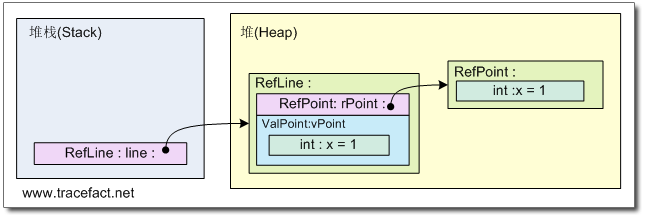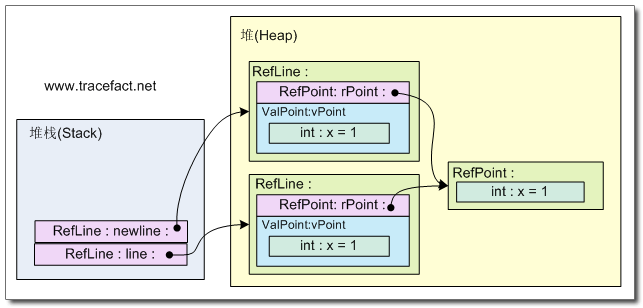ValLine newLine = line;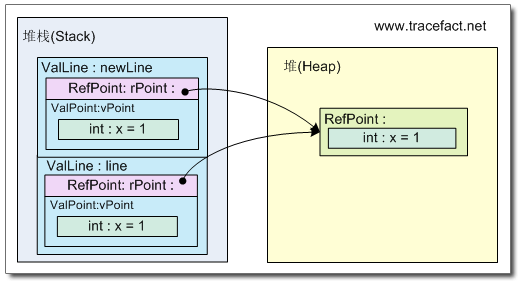public object Clone() {
return MemberwiseClone();
}

class Program {
static void Main(string[] args) {

RefPoint rPoint = new RefPoint(1);
ValPoint vPoint = new ValPoint(1);
RefLine line = new RefLine(rPoint, vPoint);

RefLine newLine = (RefLine)line.Clone();
Console.WriteLine("Original: line.rPoint.x = {0}, line.vPoint.x = {1}", line.rPoint.x, line.vPoint.x);
Console.WriteLine("Cloned: newLine.rPoint.x = {0}, newLine.vPoint.x = {1}", newLine.rPoint.x, newLine.vPoint.x);

line.rPoint.x = 10;      // 修改原先的line的 引用类型成员 rPoint
line.vPoint.x = 10;      // 修改原先的line的 值类型  成员 vPoint
Console.WriteLine("Original: line.rPoint.x = {0}, line.vPoint.x = {1}", line.rPoint.x, line.vPoint.x);
Console.WriteLine("Cloned: newLine.rPoint.x = {0}, newLine.vPoint.x = {1}", newLine.rPoint.x, newLine.vPoint.x);

}
}

Original: line.rPoint.x = 1, line.vPoint.x = 1
Cloned: newLine.rPoint.x = 1, newLine.vPoint.x = 1
Original: line.rPoint.x = 10, line.vPoint.x = 10
Cloned: newLine.rPoint.x = 10, newLine.vPoint.x = 1

### 2.深度复制

public object Clone(){       // 深度复制
RefPoint rPoint = new RefPoint();       // 对于引用类型，创建新对象
rPoint.x = this.rPoint.x;           // 复制当前引用类型成员的值 到 新对象
ValPoint vPoint = this.vPoint;          // 值类型，直接赋值
RefLine newLine = new RefLine(rPoint, vPoint);
return newLine;
}

public object Clone() {
BinaryFormatter bf = new BinaryFormatter();
MemoryStream ms = new MemoryStream();
bf.Serialize(ms, this);
ms.Position = 0;

return (bf.Deserialize(ms)); ;
}

class Program {
static void Main(string[] args) {
RefPoint rPoint = new RefPoint(1);
ValPoint vPoint = new ValPoint(2);

RefLine line = new RefLine(rPoint, vPoint);
RefLine newLine = (RefLine)line.Clone();

Console.WriteLine("Original line.rPoint.x = {0}", line.rPoint.x);
Console.WriteLine("Cloned newLine.rPoint.x = {0}", newLine.rPoint.x);

line.rPoint.x = 10;   // 改变原对象 引用成员 的值
Console.WriteLine("Original line.rPoint.x = {0}", line.rPoint.x);
Console.WriteLine("Cloned newLine.rPoint.x = {0}", newLine.rPoint.x);
}
}

Original line.rPoint.x = 1
Cloned newLine.rPoint.x = 1
Original line.rPoint.x = 10
Cloned newLine.rPoint.x = 1

[Serializable()]
public class RefPoint { /*略*/}

NOTE：关于特性(Attribute)，可以参考 .Net 中的反射(反射特性) 一文。

+ 关注